# Normalize Node

Access: NodesMathNormalize

The Normalize node takes an input vector, of any length, and normalizes it to retain its direction, but display its length as unit length which is always a value of 1.0. This normalization process is useful to describe a vector's direction without regard to its length. The following formula is used to calculate the normalized vector: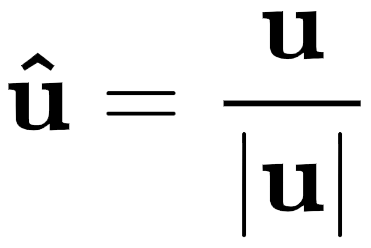Where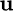is the vector [xyzw],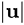is the length of the vector and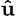represents the normalized vector.

# Normalize Node Examples

 Input Calculation Output= [RGBA] = [3 1 2 0]= 3.742R = 3 / 3.742 = 0.802G = 1 / 3.742 = 0.267B = 2 / 3.742 = 0.534A = 0 / 3.742 = 0= [0.802 0.267 0.534 0]= 1.0= [RGBA] = [10 5 4 0.5]= 11.874R = 10 / 11.874 = 0.842G = 5 / 11.874 = 0.421B = 4 / 11.874 = 0.337A = 0.5 / 11.874 = 0.042= [0.842 0.421 0.337 0.042]= 1.0= [RGBA] = [12 25 6 1]= 28.373R = 12 / 28.373 = 0.423G = 25 / 28.373 = 0.881B = 6 / 28.373 = 0.211A = 1 / 28.373 = 0.035= [0.423 0.881 0.211 0.035]= 1.0

Tip:  For more information about normalized and unit vectors, see Unit Vectors provided by Wikipedia. For information about calculating the length, or magnitude, of a vector, see Length Node.

The use of the Normalize node is specific to normal map textures, or any textures that represent a unit length vector.

The Normalize node can be used to ensure that a normal map is behaving correctly after it has been edited manually or after it has been generated from a section of the Node Graph.

# Normalize Node Inputs

 Input The vector to be normalized.# Normalize Node Outputs

 Output The normalized vector.# Normalize Node Properties

R

checkbox

When checked, the red component is included in the normalization output. When unchecked, the red component will be left out of the normalization calculation and the input red value will pass through unchanged.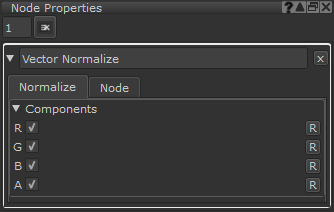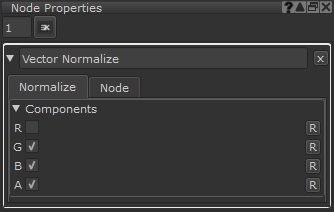RGBA are enabled so the output is: [RGBA] GBA are enabled so the output is: [RGBA]

G

checkbox

When checked, the green component is included in the normalization output. When unchecked, the green component will be left out of the normalization calculation and the input green value will pass through unchanged.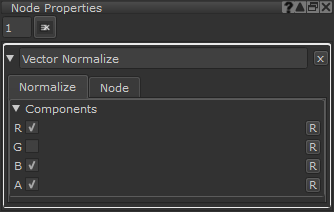RGBA are enabled so the output is: [RGBA] RBA are enabled so the output is: [RGBA]

B

checkbox

When checked, the blue component is included in the normalization output. When unchecked, the blue component will be left out of the normalization calculation and the input blue value will pass through unchanged.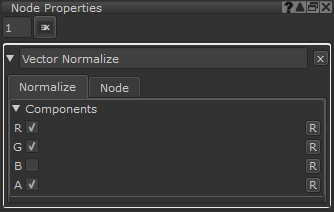RGBA are enabled so the output is: [RGBA] RGA are enabled so the output is: [RGBA]

A

checkbox

When checked, the alpha component is included in the normalization output. When unchecked, the alpha component will be left out of the normalization calculation and the input alpha value will pass through unchanged.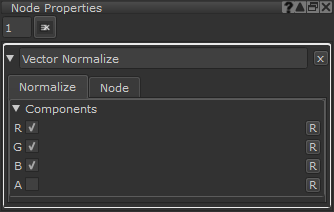RGBA are enabled so the output is: [RGBA] RGB are enabled so the output is: [RGBA]